# Coulomb's Law

### History(1)

The ancients knew that rubbing cat’s fur and amber together would allow a piece of amber to attract very light objects. In approximately 600BC Thales of Miletus observed that this rendered amber magnetic like some minerals that do not need rubbing to exhibit directional properties. He was incorrect to think these mysterious forces were the same. It is true that later science would show the forces closely related when motion is introduced into experiments, hence the word electromagnetism.

It was not until over two millennia later that English scientist William Gilbert distinguished the magnetic lodestone phenomenon from static electric attraction. For the latter, he used the word electricus meaning amber like, similar to the Greek word for amber, elektron. This latter became electric and electricity.

Swiss mathematician and physicist Daniel Bernoulli and Italian physicist Alessandro Volta in the 18th century measured attraction and repulsion using parallel plate capacitors. They may have suspected something similar to Newton’s law of gravity, dated 1686, that has an inverse square relation.

Englishman Joseph Priestley speculated the force between charges followed an inverse square law in 1767.

Scottish physicist John Robison measured the force between charged spheres as varying according to 1/(r)2.06 in 1769.

English chemist Henry Cavendish investigated force depending on charge and distance, but did not publish.

French physicist Charles-Augustin de Coulomb in 1785 published three papers on electricity and magnetism containing his law, Coulomb’s Law.

### Coulomb’s Law(2)(3)

Coulomb experimentally defined electric charge in terms of force. He showed that two charged particles, or small objects, opposed each other in inverse proportion to the square of the distance between them. This was done in air which closely approximates vacuum for this propose. With a proportionality constant Coulombs law is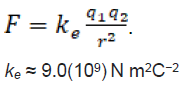(1)
where N – Newtons, m – meters, C – Coulombs.

A convenient standard for charge is the electron. This becomes the unit of charge called the Coulomb sometimes written C, q, or Q as a dimension symbol. C is used here so q can be used as a quantity of charge. One electron charge is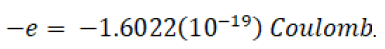This value is set to agree with other electrical units. The proportionality constant is defined to make force be in Newtons. ke is further defined as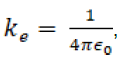where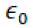is called the permittivity of free space.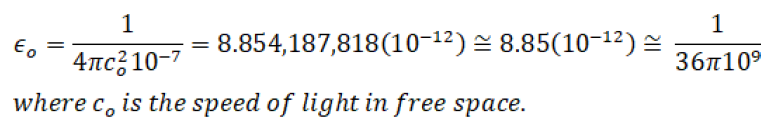4𝞹 is inserted to simplify Maxwell’s equations. It is interesting to note that in 1983 the meter was redefined to make the speed of light exactly 299,792,458 meters per second.(4)

When magnetics is introduced,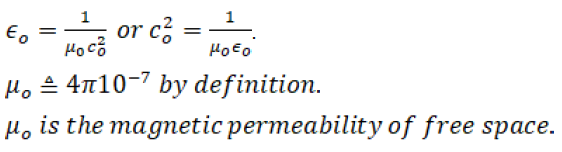This is known as the rationalized MKSC units. This indicates that the Coulomb is the fourth fundamental unit. All other units are secondary to meter-kilogram-second-Coulomb and may be defined in terms of these fundamental units.

Finally Coulomb’s law may be given as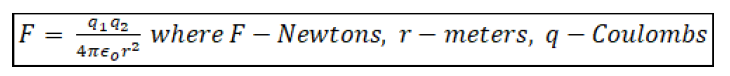(2)

For an intuitive insight to what one Coulomb of charge represents, consider two point charges of 1Coulomb placed 1 meter apart. The force between would be approximately 9(109) Newtons or approximately one million (106) tons (0.2248 lbf per Newton). That is a big charge!

Note that to make the equation yield Newtons,must have the units of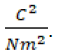. If a Farad is defined as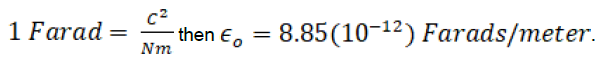The relation can be expressed in vector form as follows.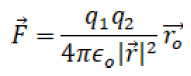It should be noted that this is an electrostatic approximation. Quantum field theory requires the introduction of magnetic considerations. This in turn uses Einstein’s theory of relativity.

### References

1. https://en.wikipedia.org/wiki/Coulomb’s_law
2. Allen Nussbaum, “Electromagnetic and Quantum Properties of Materials,” Prentice-Hall, 1966
3. John D. Kraus, “Electromagnetics”, McGraw Hill, 1953
4. https://en.wikipedia.org/wiki/Speed_of_light

Top